# TransferFunction

Complex Transfer Function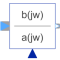# Information

This information is part of the Modelica Standard Library maintained by the Modelica Association.

The complex input u is multiplied by the complex transfer function (depending on frequency input w) to obtain the complex output y (nb = dimension of b, na = dimension of a):

```           b*(jw)^[nb-1] + b*(jw)^[nb-2] + ... + b[nb]
y(jw) = ------------------------------------------------- * u(jw)
a*(jw)^[na-1] + a*(jw)^[na-2] + ... + a[na]
```

# Parameters (3)

useConjugateInput Value: false Type: Boolean Description: If true, input is processed conjugate complex Value: {1} Type: Real[:] Description: Numerator coefficients of transfer function (e.g., 2*s+3 is specified as {2,3}) Value: {1} Type: Real[:] Description: Denominator coefficients of transfer function (e.g., 5*s+6 is specified as {5,6})

# Connectors (3)

u y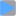Type: ComplexInput Description: Connector of Complex input signal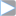Type: ComplexOutput Description: Connector of Complex output signal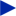Type: RealInput Description: Frequency input

# Components (5)

uInternal bw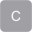Type: Complex Description: Equals either u or conjugate complex input u if useComplexInput = trueType: Complex[size(b, 1)]Type: Complex[size(a, 1)]Type: ComplexType: Complex

# Used in Examples (1)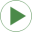ShowTransferFunction Modelica.ComplexBlocks.Examples Test Complex Transfer Function Block